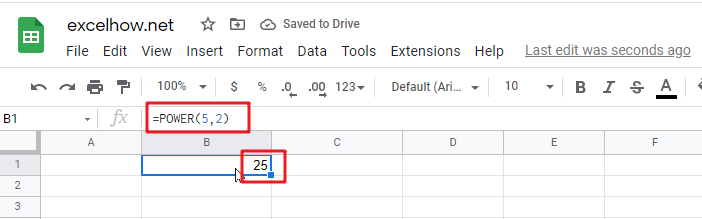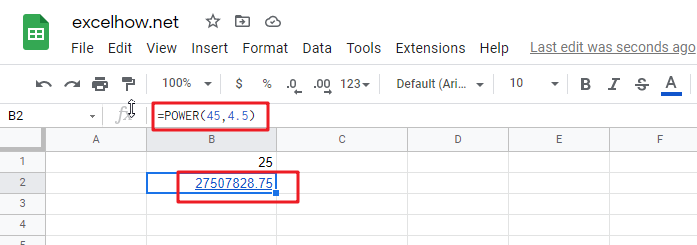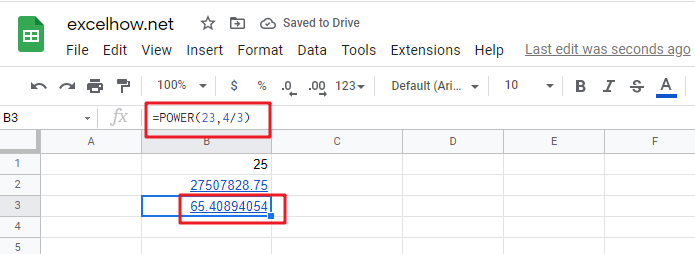# ExcelHow

This post will guide you how to use Google Sheets POWER function with syntax and examples in Google Spreadsheets.

## Description

The Google Sheets POWER function returns the result of a number raised to a given power. So you can use the POWER function to calculate a given number raised to a supplied power in Google Sheets.

The POWER function is a build-in function in google spreadsheets and it is categorized as a Math and Trigonometry Function.

## Syntax

The syntax of the POWER function is as below:

`= POWER (number,power)`

Where the POWER function arguments are:

• `number`This is a required argument. The base number that you want to raise to a power
• `Power` This is a required argument. The exponent to which the base number is raised.

Note:

• The “^” operator can be used instead of POWER to indicate to what power the base number is to be raised.

## Google Sheets POWER Function Examples

The below examples will show you how to use Google Sheets POWER Function to calculate the result of a number raised to a power.

1# get the result of 5 squared, enter the following formula in Cell B1.

`=POWER(5,2)`2# get the result of number 45 raised to the power of 4.5, enter the following formula in Cell B2.

`=POWER(45,4.5)`3# get the result of number 23 raised to the power of 4/3, enter the following formula in Cell B3.

`=POWER(23,4/3)`